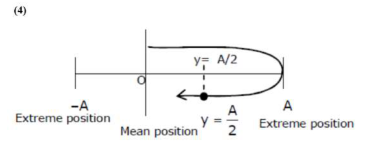# if`
Question:

$Y=A \sin \left(\omega t+\phi_{0}\right)$ is the time - displacement equation of a SHM, At $t=0$ the displacement of the particle is $Y=\frac{A}{2}$ and it is moving along negative $x$-direction. Then the initial phase angle $\phi_{0}$ will be

1. (1) $\frac{\pi}{6}$

2. (2) $\frac{\pi}{3}$

3. (3) $\frac{2 \pi}{3}$

4. (4) $\frac{5 \pi}{6}$

Correct Option: , 4

Solution:The initial phase angle $\phi_{0}=\pi-\frac{\pi}{6}$

$=\frac{5-\pi}{6}$